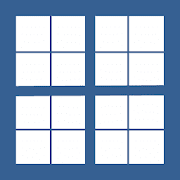# MontCalc Pro (Time Calculator)Everyone
TIME CALCULATOR
★ How many days are there when I or someone was born until today?
★ How many days are there from the beginning of the year, or from a certain date, until today?
★ How many days have passed and how many days are left until the end of the year?
★ How many days are there between the number of days that have passed when doubled? (chronological symmetry of the year).
★ Separate the year or any number of days from a date, showing the date where each period begins and ends, including the day of the week.
★ Automatically separate by common dividers the year or any number of days by periods that show the start and end date, including the day of the week.
★ Set two or more dates and find out how many calendar days are between them and the grand total. (Start and end of an event, for example).
★ Simulate chronological results by automatically advancing and rewinding the days and months of the year as if you were in the past and the future.
★ Visualize the sequence of the 52 weeks of the year (beginning and end with the day of the week) by setting 364+1 or 364+2 and dividing the first period by 52.
★ Set a date, a number of calendar days and know when this period will end, including the day of the week. (vacation period, for example).
★ Let your imagination run wild and have fun with this calculator, which can make you stop in time ... or "travel" on it.

PRESENTATION
The MontCalc calculator is focused on alphanumeric calculations, period-of-days, dates, and conversion of numbering systems. It also includes basic and advanced mathematical operations.

1-MATHEMATICAL MODULE:
★ Arithmetic operations,
★ Calculation of Square and Cubic Root,
★ Calculation of Percentages,
★ Calculation of Potentiation and Factoring,
★ Calculates the Sum of a number,
★ Calculates the Maximum Common Divider and Minimum Common Multiple,
★ Convert Roman to Decimal and vice versa,
★ Convert Decimal System to Binary and Hexadecimal.
★ Convert Binary System to Decimal and Hexadecimal.
★ Convert Hexadecimal to Decimal and Binary.

2-MODULE OF TIME
★ Calculate past and missing days to end the year.
★ Calculate the symmetry of the past or future days of the year.
★ Calculate from the current day how many have passed and are missing by the end of the year.
★ Calculates and displays the start and end date of user defined periods.
★ Stopwatch with multiple registration of terms of the same event.

3-ALPHANUMERIC MODULE
★ Calculate the value of words according to the ALPHANUMERIC SYSTEM METHODIZED by the author of MontCalc.

4-DATA MODULE
★ Calculate the number of days between two or more dates.

LAYOUT
★ Set Colors, Borders, Shadows, and Reveal effect to the calculator.
★ Choose the Zoom Level that best suits your device.
★ Use the compact mode showing one module at a time.

EXTRAS
★ Very useful table of quivalencies between decimal, hexadecimal, alphanumeric, roman and summation systems, up to 255.
★ Extensive Help material on how to operate the calculator.
★ A super cool puzzle with eight sorts of sorts.

PAINT
★ Includes MontCell Paint Canvas tool.
★ Drag and drop, resize and rotate letters and graphic objects on the screen.

ATTENTION!
To return MMC, MDC, and EXP, read the manual for calculation rules.

MontCalc Calculator
You in Digital Mode
https://montcalc.com

Série Manancial
Collapse

Review Policy

## What's New

Interface Improvements and Usability.
Collapse

Eligible for Family LibraryEligible if bought after 7/2/2016. Learn More
Updated
March 28, 2020
Size
816k
Installs
10+
Current Version
4.0
Requires Android
4.4 and up
Content Rating
Everyone
Permissions
Offered By
Série Manancial
Developer